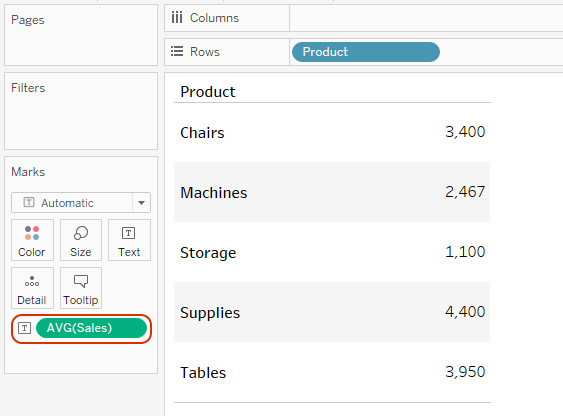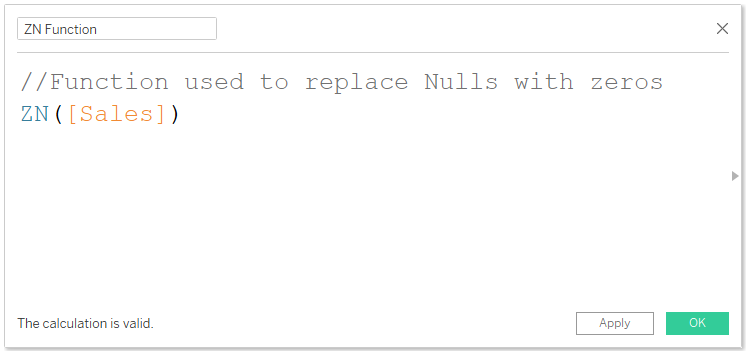top of page
Search
• Bernard K

# Using the ZN Function in TableauThe ZN function when used in a calculation returns the default values if it is not nulls, otherwise it returns zeros. In summary, we can say that we use the ZN function to replace null values with zeros within our computations.

Syntax

ZN (Expression) – this function returns the expression if its not null, otherwise returns zero.

For example

This sample dataset shows the sales for different products in different quarters.If you were to compute the average sales per product, this is what you’ll get.(And the reason you’re getting such results - is because NULL values are ignored when computing the average)

But suppose instead, you want to replace the NULL values in your computations with zeros. You can do that using the ZN function as shown below.Notice this calculation replaces NULLs with zeros as you can see when you view the data.And now using this calculation to compute the average leaves us with.(From the resulting view, you can see the difference between the two computations – as highlighted by the star shapes.)

Therefore, next time you need to convert a large list of nulls into zeros, don’t forget the ZN function.